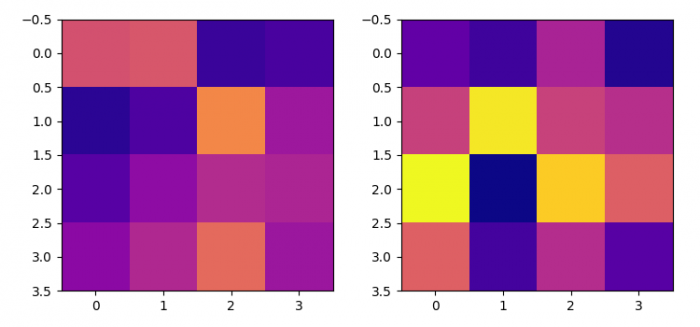# Set two Matplotlib imshow plots to have the same colormap scale

MatplotlibPythonData Visualization

#### Python Data Science basics with Numpy, Pandas and Matplotlib

Most Popular

63 Lectures 6 hours

#### Data Visualization using MatPlotLib & Seaborn

11 Lectures 4 hours

#### MatPlotLib with Python

9 Lectures 2.5 hours

To set two matplotlib imshow() plots to have the same colormap scale, we can take the following

## Steps

• Set the figure size and adjust the padding between and around the subplots.
• Create d1 and d2 matrices using Numpy.
• Get the resultant matrix to get the maximum and minmum value.
• Use amin and amax methods for minimum and maximum values.
• Create a new figure or activate an existing figure.
• Add an '~.axes.Axes' to the figure as part of a subplot arrangement, with nrows=1, ncols=2 at index 1
• Using imshow() method with vmin and vmax, define the data range that the colormap covers.
• Repeat steps 6 and 7 with data
• To display the figure, use show() method.

## Example

import numpy as np
from matplotlib import pyplot as plt

plt.rcParams["figure.figsize"] = [7.50, 3.50]
plt.rcParams["figure.autolayout"] = True

data1 = np.random.randn(4, 4)
data2 = np.random.randn(4, 4)

resultant = np.array([data1, data2])
min_val, max_val = np.amin(resultant), np.amax(resultant)
fig = plt.figure()

ax = fig.add_subplot(1, 2, 1)
ax.imshow(data1, cmap="plasma", vmin=min_val, vmax=max_val)

ax2 = fig.add_subplot(1, 2, 2)
ax2.imshow(data2, cmap="plasma", vmin=min_val, vmax=max_val)

plt.show()

## Output